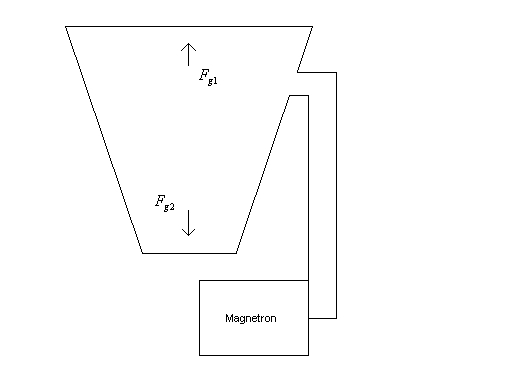SPR Ltd.

 Home Background Benefits Theory Development Applications Future FAQ

Theory

Principle of Operation

At first sight the idea of propulsion without propellant seems impossible. However the technology is firmly anchored in the basic laws of physics and following an extensive review process, no transgressions of these laws have been identified.

The principle of operation is based on the well-known phenomenon of radiation pressure. This relies on Newton’s Second Law where force is defined as the rate of change of momentum. Thus an electromagnetic (EM) wave, travelling at the speed of light has a certain momentum which it will transfer to a reflector, resulting in a tiny force.

If the same EM wave is travelling at a fraction of the speed of light, the rate of change of momentum, and hence force, is reduced by that fraction. The propagation velocity of an EM wave, and the resulting force it exerts, can be varied depending on the geometry of a waveguide within which it travels. This was demonstrated by work carried out in the 1950’s. (CULLEN, A.L. ‘Absolute Power Measurements at Microwave Frequencies’ IEE Proceedings Vol 99 Part 1V 1952 P.100)

Thus if the EM wave travelling in a tapered waveguide is bounced between two reflectors, with a large velocity difference at the reflector surfaces, the force difference will give a resultant thrust to the waveguide linking the two reflectors. If the reflectors are separated by a multiple of half the effective wavelength of the EM wave, this thrust will be multiplied by the Q of the resulting resonant cavity, as illustrated in fig 1.Fig 1. Diagram of an engine concept.

The inevitable objection raised, is that the apparently closed system produced by this arrangement cannot result in an output force, but will merely produce strain within the waveguide walls. However, this ignores Einstein’s Special Law of Relativity in which separate frames of reference have to be applied at velocities approaching the speed of light. Thus the system of EM wave and waveguide can be regarded as an open system, with the EM wave and the waveguide having separate frames of reference.

A similar approach is necessary to explain the principle of the laser gyroscope, where open system attitude information is obtained from an apparently closed system device.